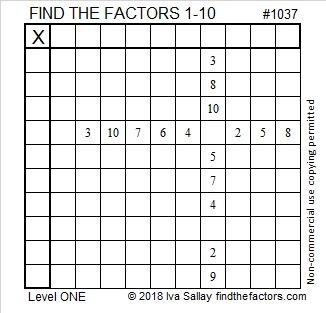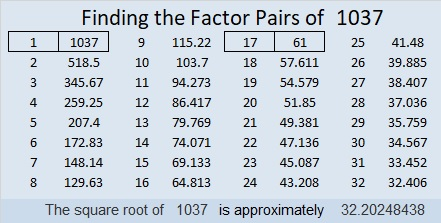# 1037 and Level 1

This week I’ll start with this level 1 puzzle that is just about as easy as they get. If you’ve never done a Find the Factors puzzle, you can still easily figure this one out. The finished puzzle will look like a regular multiplication table but with the factors in a different order than usual. Go ahead, give this one a try!Print the puzzles or type the solution in this excel file: 10-factors-1035-1043

Now I’ll write some facts about the number 1037:

• 1037 is a composite number.
• Prime factorization: 1037 = 17 × 61
• The exponents in the prime factorization are 1 and 1. Adding one to each and multiplying we get (1 + 1)(1 + 1) = 2 × 2 = 4. Therefore 1037 has exactly 4 factors.
• Factors of 1037: 1, 17, 61, 1037
• Factor pairs: 1037 = 1 × 1037 or 17 × 61
• 1037 has no square factors that allow its square root to be simplified. √1037 ≈ 32.202481037 is palindrome 191 in BASE 28 because 28² + 9(28) +1 = 1037

1037 is the sum of two squares two different ways:
29² + 14² = 1037
26² + 19² = 1037

1037 is the hypotenuse of FOUR Pythagorean triples:
187-1020-1037 which is 17 times (11-60-61)
315-988-1037 calculated from 26² – 19², 2(26)(19), 26² + 19²
488-915-1037 which is (8-15-17) times 61
645-812-1037 calculated from 29² – 14², 2(29)(14), 29² + 14²

This site uses Akismet to reduce spam. Learn how your comment data is processed.